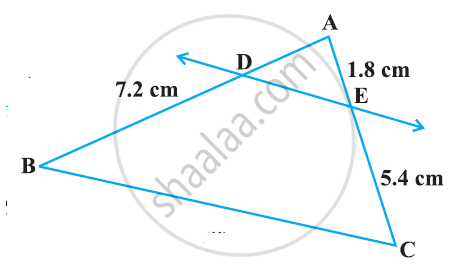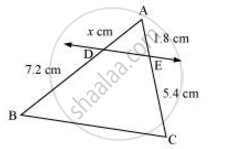# See the given Figure.  DE || BC. Find AD - Mathematics

See the given Figure.  DE || BC. Find AD#### SolutionIt is given that DE || BC.

By using basic proportionality theorem, we obtain

(AD)/(DB)=(AE)/(EC)

x/7.2=1.8/5.4

x = (1.8xx7.2)/5.4

x = 2.4

Concept: Similarity of Triangles
Is there an error in this question or solution?

#### APPEARS IN

NCERT Class 10 Maths
Chapter 6 Triangles
Exercise 6.2 | Q 1.2 | Page 128